Next: Poiseuille Flow Up: Incompressible Viscous Flow Previous: Flow Between Parallel Plates

# Flow Down an Inclined Plane

Consider steady, two-dimensional, viscous flow down a plane that is inclined at an angleto the horizontal. Letmeasure distance along the plane, and letbe a transverse coordinate such that the surface of the plane corresponds to. Suppose that the fluid forms a uniform layer of depthcovering this surface. (See Figure 10.2.)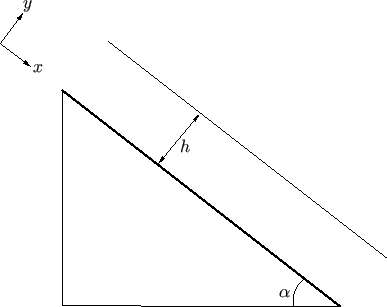The generalized pressure gradient within the fluid is written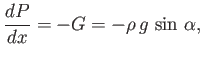(10.12)

where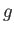is the acceleration due to gravity. In this case, there is no gradient in the actual pressure in the-direction, and the flow down the plane is driven entirely by gravity. As before, we can write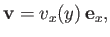(10.13)

and Equation (10.2) again reduces to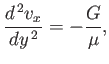(10.14)

where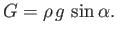(10.15)

Application of the no slip condition at the surface of the plane,, yields the standard boundary condition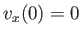. However, the appropriate physical constraint at the fluid/air interface,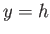, is that the normal viscous stress there be zero (i.e.,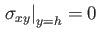), because there is nothing above the interface that can exchange momentum with the fluid (assuming that the finite inertia and viscosity of air are both negligible.) Hence, from Section 1.18, we get the boundary condition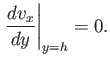(10.16)

The solution to Equation (10.14) that satisfies the boundary conditions is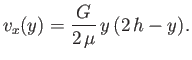(10.17)

Thus, the profile is again parabolic. In fact, it is the same as the lower half of the profile obtained when fluid flows between two (stationary) parallel plates situated a perpendicular distance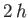apart.

The net volume flux (per unit width in the-direction) of fluid down the plane is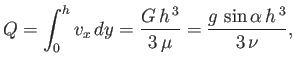(10.18)

where use has been made of Equation (10.15). Here,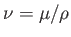is the kinematic viscosity of the fluid. Thus, given the rate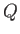that fluid is poured down the plane, the depth of the layer covering the plane becomes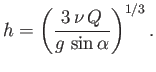(10.19)

Suppose that the rate at which fluid is poured down the plane is suddenly increased slightly fromto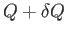. We would expect an associated change in depth of the layer covering the plane fromto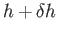, where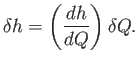(10.20)

Let the interface between the layers of different depth propagate in the-direction at the constant velocity. In a frame of reference that co-moves with this interface, the volume fluxes (per unit width) immediately to the right and to the left of the interface are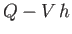and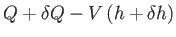, respectively. However, in a steady state, these fluxes must equal one another. Hence,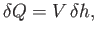(10.21)

or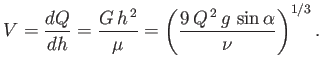(10.22)

As can easily be verified, this velocity is twice the maximum fluid velocity in the layer.Next: Poiseuille Flow Up: Incompressible Viscous Flow Previous: Flow Between Parallel Plates
Richard Fitzpatrick 2016-03-31